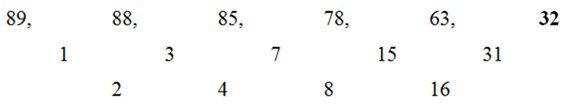# RRB Clerk Prelims Quantitative Aptitude (Day-01)

Dear Aspirants, Our IBPS Guide team is providing new series of Quantitative Aptitude Questions for RRB Clerk 2020 Prelims so the aspirants can practice it on a daily basis. These questions are framed by our skilled experts after understanding your needs thoroughly. Aspirants can practice these new series questions daily to familiarize with the exact exam pattern and make your preparation effective.

Start Quiz

Data Interpretation

Directions (Q. 1- 5) Study the following bar graph and table carefully to answer the questions given below:

The following bar graph shows data related to population of different states(in lakhs) in the year 1992.The following table shows the ratio between male to female and literate to illiterate and graduates to under graduates.1) If in the year 1993, the population of AP is increased by 10 % and the population of Bihar is increased by 12 % compared to the previous year, then find the ratio of the population of AP to that of Bihar in the year 1993?

A.521 : 540

B.405 : 530

C.408 : 505

D.407 : 560

E.None of these

2) The total female population in AP is approximately what percentage of total female population in HP?

A.90 %

B.110 %

C.120 %

D.140 %

E.85 %

3) If 70% of total number of literate population in Assam is graduate, then find the total number of under graduates in the Assam in the year 1992?

A.65300

B.70000

C.62000

D.82100

E.None of these

4) In Haryana, If 70% of the females are literate and 75% of the males are literate, then find the total number of illiterates in the state?

A.122500

B.95000

C.84000

D.76000

E.None of these

5) Find the ratio between the total number of literate population in Assam to that of total number of literate population in Bihar?

A.2 : 5

B.3 : 5

C.7 : 3

D.2 : 3

E.None of these

Missing Number Series

Directions (Q. 6 – 10): What should come in place of question mark (?) in the following questions?

6) 5, 28, 47, 64, 77, ?

A.96

B.98

C.85

D.106

E.88

7) 6, 4, 5, 11, 39, ?

A.160

B.193

C.189

D.183

E.171

8) 29, 15, 16, 34, 140, ?

A.1335

B.1026

C.1128

D.1129

E.1600

9) 89, 88, 85, 78, 63, ?

A.32

B.30

C.34

D.27

E.23

10) 190, 94, 46, 22, ?, 4

A.16

B.10

C.9

D.13

E.8

Required ratio = (370000*(110/100)) : (500000*(112/100))
= > 407 : 560

The female population in AP = 370000*(6/10) = 222000
The female population in HP = 280000*(4/7) = 160000
Required percentage = (222000/160000)*100 = 140 %

The total no of literate under graduates population in Assam= 300000*(7/9)*(30/100)
= > 70000

If 70% of the females are literate and 75% of the males are literate
Female illiterate Haryana = (450000*4/9)*(30/100)
= > 60000
Male illiterate Haryana = (450000*5/9)*(25/100)
= > 62500
Total number of illiterate population in Haryana =122500

Required ratio = (300000*(7/9)) : (500000*(1/5))
= > 7 : 3

The pattern is, +23, +19, +17, +13, +11

The pattern is, *1 – 2, *2 – 3, *3 – 4, *4 – 5, *5 – 6

The pattern is, *0.5 + 0.5, *1 + 1, *2, +2, *4 + 4, *8 + 8The difference of difference is, *2